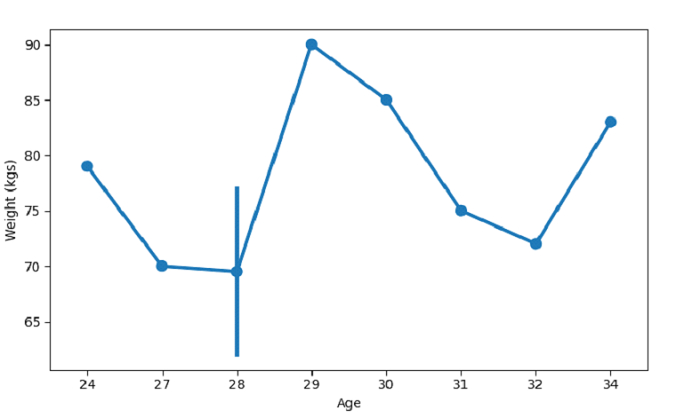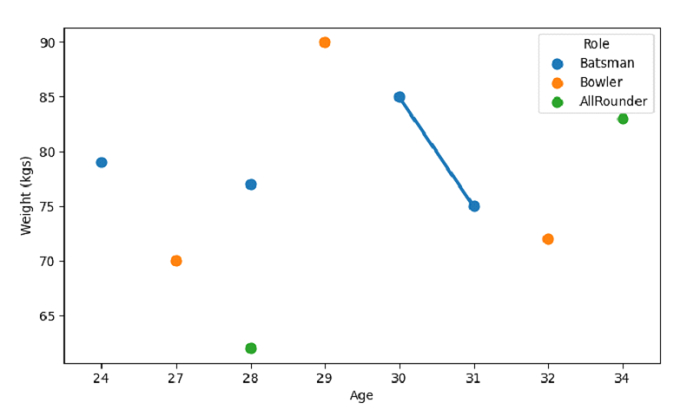# Create a Point plot with SeaBorn â€“ Python Pandas

The seaborn.pointplot() is used to create a point plot. Let’s say the following is our dataset in the form of a CSV file − Cricketers.csv

At first, import the required 3 libraries −

import seaborn as sb
import pandas as pd
import matplotlib.pyplot as plt

Load data from a CSV file into a Pandas DataFrame −

dataFrame = pd.read_csv("C:\Users\amit_\Desktop\Cricketers.csv")


Plotting point plot with Age and Weight column −

sb.pointplot(x =dataFrame["Age"], y = dataFrame["Weight"], data = dataFrame)

## Example

Following is the code −

import seaborn as sb
import pandas as pd
import matplotlib.pyplot as plt

# Load data from a CSV file into a Pandas DataFrame

# plotting point plot with Age and Weight column
sb.pointplot(x =dataFrame["Age"], y = dataFrame["Weight"], data = dataFrame)

# label
plt.ylabel("Weight (kgs)")

# display
plt.show()

## Output

This will produce the following output −## Example

Let us see another example wherein we have set the hue parameter −

import seaborn as sb
import pandas as pd
import matplotlib.pyplot as plt

# Load data from a CSV file into a Pandas DataFrame

# plotting point plot with Age and Weight column
# we have set the hue parameter as Role columns
sb.pointplot(dataFrame['Age'],dataFrame['Weight'], hue=dataFrame['Role'])

# label
plt.ylabel("Weight (kgs)")

# display
plt.show()

## Output

This will produce the following output −